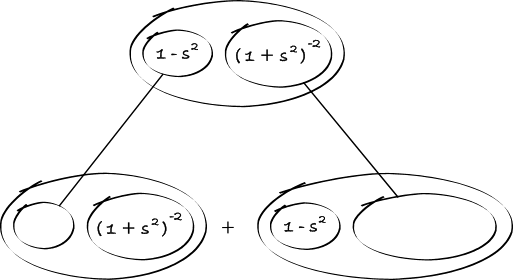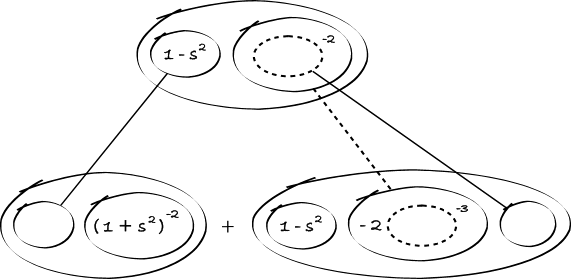# Thread: Rel. Max and Rel Min, going wrong in my second derivative

1. ## Rel. Max and Rel Min, going wrong in my second derivative

here's the problem, f(x)= s/1+s^2

I've been staring at this problem for almost 30 minutes and i can't see where i go wrong on my second derivative...i've already gotten my critical points for f'(x) which are -1 and 1

f'(x)= 1-s^2/(1+s)^2
f"(x)= 1-s^2/(1+s)^2 = (1+s)^2(-2s)-(1-s2)(2)(1+s)/(1+s)^4 ...cancel my (1+s) = (1+s)(-2s)-(1-s^2)(2)/(1+s)^3 =-2s-2s^2-2+2s^2/(1+s)^3 Ultimately getting -2(s+1)/(1+s)^3

2.Originally Posted by larasanuthere's the problem, f(x)= s/1+s^2

I've been staring at this problem for almost 30 minutes and i can't see where i go wrong on my second derivative...i've already gotten my critical points for f'(x) which are -1 and 1

f'(x)= 1-s^2/(1+s)^2
f"(x)= 1-s^2/(1+s)^2 = (1+s)^2(-2s)-(1-s2)(2)(1+s)/(1+s)^4 ...cancel my (1+s) = (1+s)(-2s)-(1-s^2)(2)/(1+s)^3 =-2s-2s^2-2+2s^2/(1+s)^3 Ultimately getting -2(s+1)/(1+s)^3
Your expression for f'(s) is wrong (and the variable is s, not x. Or should it be x and not s ....?).

It should be f'(s) = (1-s^2)/(1+s^2)^2. No doubt this will affect the calculation for f''(s).

And note the red stuff. Lack of attention to detail is the leading cause of mistakes. If you're not using latex, use brackets to avoid ambiguity in what you post (and in what you input to a calculator ....).

3. so, g'(s)= 1-s^2/(1+s^2)^2
g"(s)= 2s(s^2-3)/(1+s^2)^3

i'm still on that same problem, can u work out the second derivative please

4.Originally Posted by larasanutso, g'(s)= 1-s^2/(1+s^2)^2
g"(s)= 2s(s^2-3)/(1+s^2)^3

i'm still on that same problem, can u work out the second derivative please
No, I'm not going to work it out. That's your job. Apply the quotient rule and simplify the numerator. Do all the steps - carefully. Show all your working and I'll point out the mistakes (probably more careless ones).

5. Drawing a picture may help you to take the necessary care. Note that the quotient rule results from the product rule, and in some cases - like the second differentiation here - you may have less simplifying to do afterwards if you apply the product rule directly.

The product rule tells you to differrentiate (with respect to s) down the straight lines only, in this pattern:Zooming in further... the chain rule tells you to differentiate down the straight dashed line with respect to the inner function i.e. with respect to the dashed balloon:(Then multiply out the balloons as brackets and make (1 + s^2)^3 a common denominator.)

Hope this helps. Use it for integration too - see Balloon Calculus: worked examples from past papers.

#### Search Tags

derivative, max, min, rel, wrong